Zhao, C.-S., Zuo, P., Wang, X., He, Y.-Z., Chen, H.-T., Zhang, Y., and Li, L.-H. (2022). "Parameter optimization of a biodegradable agricultural film manufactured with wheat straw fiber," BioResources 17(2), 2331-2346.

#### Abstract

Straw fiber mulch film is a promising alternative to petroleum-based plastic mulching film. The optimal combination of process parameters during the manufacturing process of the wheat straw fiber-based plant fiber film (hereinafter referred to as film), was studied via a five-factor and five-level central composite design methodology. Response surface methodology was used to analyze the effects of sizing agent content, wet strength agent mass fraction, basis weight, KP pulp mixing ratio, and beating degree of wheat straw fiber on the dry tensile index, wet tensile index, and degradation time. The optimal parameters were as follows: a sizing agent content of 2.0%, a wet strength agent mass fraction of 60%, a beating degree of wheat straw fiber of 60 °SR, a basis weight of 54.6 to 60.8 g/m2, and a KP pulp mixing ratio of 37.6% to 44.7%. These parameters achieved the desired physical properties, i.e., a degradation time of 55 to 65 d, a dry tensile index of 10 to 12 N·m/g, and a wet tensile index 3.5 to 5.5 N·m/g. With the optimal manufacturing parameters, straw mulch film would meet the demands for agriculture.

Parameter Optimization of a Biodegradable Agricultural Film Manufactured with Wheat Straw Fiber

Cheng-Shun Zhao,a Peng Zuo,b Xing Wang,a Yu-Ze He,c Hai-Tao Chen,a Ying Zhang,a,* and Long-Hai Li a,*

Straw fiber mulch film is a promising alternative to petroleum-based plastic mulching film. The optimal combination of process parameters during the manufacturing process of the wheat straw fiber-based plant fiber film (hereinafter referred to as film), was studied via a five-factor and five-level central composite design methodology. Response surface methodology was used to analyze the effects of sizing agent content, wet strength agent mass fraction, basis weight, KP pulp mixing ratio, and beating degree of wheat straw fiber on the dry tensile index, wet tensile index, and degradation time. The optimal parameters were as follows: a sizing agent content of 2.0%, a wet strength agent mass fraction of 60%, a beating degree of wheat straw fiber of 60 °SR, a basis weight of 54.6 to 60.8 g/m2, and a KP pulp mixing ratio of 37.6% to 44.7%. These parameters achieved the desired physical properties, i.e., a degradation time of 55 to 65 d, a dry tensile index of 10 to 12 N·m/g, and a wet tensile index 3.5 to 5.5 N·m/g. With the optimal manufacturing parameters, straw mulch film would meet the demands for agriculture.

DOI: 10.15376/biores.17.2.2331-2346

Keywords: Central composite design; Mulch; Wheat straw; Optimization; Degradable

Contact information: a: College of Engineering, Northeast Agricultural University, No. 600 Changjiang Road, Xiangfang District, Harbin, Heilongjiang Province 150030 China; b: College of Arts and Sciences, Northeast Agricultural University, No. 600 Changjiang Road, Xiangfang District, Harbin, Heilongjiang Province 150030 China; c: College of International Exchange, Northeast Agricultural University, No. 600 Changjiang Road, Xiangfang District, Harbin, Heilongjiang Province 150030 China; 1: The first two authors contributed equally to this work, and the corresponding authors contributed equally to this paper;

* Corresponding authors: lilonghai@neau.edu.cn; zhangying0604@163.com

INTRODUCTION

With low biodegradation rates and long degradation times, the plastic residues left in farmlands after plastic film recycling have remained in the soil in the form of micro-plastics and nano-plastics, leading to the destruction of the structure of the soil, which reduces total grain output, as the plastic residues hinder nutrition absorption behavior (Tokiwa et al. 2009 Jambeck et al. 2015; He et al. 2018; Ng et al. 2018; Kumar et al. 2020). To solve this problem, researchers have developed new environmental-friendly films with completely biodegradable materials, e.g., polylactic acid plastic (Yu et al. 2019), biomass-based environmentally friendly plastics (Kyrikou et al. 2011; Corti et al. 2010; Xia et al. 2021), enzyme-based plastics (Lu et al. 2004; DelRe et al. 2021), etc. Straw fiber-based degradable films are a new agricultural film which has great potential to replace plastic films, as it is environmental-friendly, biodegradable, and has renewable properties (Haapala et al. 2014, 2015).

The straw film is fully biodegradable, such that it does not adversely affect the soil and environment. In addition, the degraded film would become an organic fertilizer, which could make the soil more fertile as well as balance the nutrients. Mechanical treatment technology is widely used as a green and environmental friendly fibrillation treatment technology. In this study, the specific structure of the screw used for processing the material will continuously cause the water-containing material to undergo a fixed-axis spiral propulsion motion, and be forcibly sheared at several shear ports through which the size is much smaller than the initial material size. This shear force forces the material to change from a conduit state to a fragmented state, and after shearing at multiple shear ports, the fiber is broomed into slender fiber branches, which are suitable for biomass mulch to build a stable building structure. The fiber film formed by the fiber-to-fiber build-up structure alone is not mechanically suitable for farm usage, so the team added the same chemical additives to the raw material as are employed in the traditional paper-making process. In addition, the same molding technique as the paper making process is used to prepare the fiber film (Zhang et al. 2014, 2020a,b, 2021; Maraveas 2020).

The properties of water vapor permeability, heat preservation, and soil moisture conservation have been heavily studied. It has been shown that wheat straw fiber film can effectively stabilize the moisture in the soil and increase the temperature needed for crops. Due to the impermeability of the film, it can effectively suppress the stressing effect of weeds around the crop (Fu et al. 2008; Han et al. 2011; Chen et al. 2018; Ming et al. 2019; Maraveas 2019). With a reported wheat production of 4,303,200 tons in 2021, the large amount of wheat straw provides abundant raw materials for biomass fiber mulch. Secondly, the unique tubular structure of wheat straw ensures the effective production length of the fibers, which is helpful for the mechanical strength of the fiber mulch, so the fiber mulch produced from wheat straw has certain advantages in comprehensive performance compared to other fiber mulches. Unlike other single tests to evaluate the performance of fiber mulch, this study couples the dry and wet tensile strength and degradation cycle of fiber mulch through a multi-faceted and multi-combination test protocol. The on-farm use of wheat fiber mulch was evaluated from two perspectives: use performance and ecological degradation. Therefore, in this study, a new plant film made from wheat straw was assessed, and a central composite design (CCD) methodology was employed to design the processing experiments. To optimize the best combination of processing variables, the response surface methodology (RSM) was adopted to optimize the processing variables that affected the degradation time, dry tensile strength, and wet tensile strength of wheat straw mulching film.

EXPERIMENTAL

Materials

Longchun 166 wheat straw was harvested from the Heilongjiang province of China in June 2020. In addition, unbleached kraft (KP) pulp fiber, a sizing agent (at a concentration of 20 g/L), a wet strength agent (at a concentration of 20 g/L), and a dry strength agent (at a concentration of 20 g/L) were used, as shown in Table 1.

Table 1. Reagents Required for the Test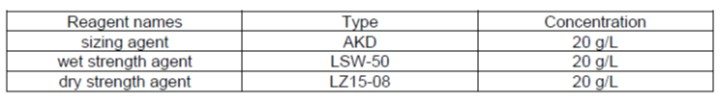Equipment

Various devices were used in the experiments as follows: a D200 straw pretreatment machine (a 25 ℃ to 120 ℃ working temperature and a 0 r/min to 140 r/min processing speed) developed by Northeast Agricultural University of China, Harbin, China; a ZT4-00 Valley Hollander beater (Zhongtong Test Equipment Co., Ltd., Xingping, China); a ZJG-100 Schopper’s beating degree instrument (Changchun Yueming Scientific Instruments Co., Ltd., Changchun, China); a ZCX-A manual sheet former (Changchun Yueming Scientific Instruments Co., Ltd., Changchun, China); a DRK101A paper product quality detector (Jinan Nake Industry and Trade Co., Ltd., Jinan, China); a JA5003B electronic balance with 0.001 g precision (Shanghai Jingke Tianmei Scientific Instruments Co., Ltd., Shanghai, China); a straw rubbing and cutting machine (Harbin Longmu Machinery Company, Harbin, China); a RPSY-4 electronic constant temperature water bath pot with 0.1 °C precision (Tester Instrument Co., Ltd, Tianjin, China); and a YHG400-BS far infrared fast constant temperature drying box (Yuejin Medical Device Co., Ltd, Shanghai, China).

Methods

The study involved five factors and five levels, so the central composite involved (CCD) method was used to design the manufacturing process experiments to investigate the effect of a single influencing factor on the film properties and their interaction effect. The five influencing factors considered were as follows: the sizing agent content (X1), the wet strength agent mass fraction (X2), the basis weight (X3), the KP pulp mixing ratio (X4), and the beating degree of wheat straw fiber (X5). The dry tensile index (y1), wet tensile index (y2), and degradation time (y3) were selected as indicators. The ranges and levels of the independent factors were shown in Table 2.

Firstly, Longchun 166 rice with 17% moisture content is taken as raw material, and the semi-finished product with average length of 132 mm and 83% kneading degree was obtained by kneading and cutting machine (the feeding capacity of kneading and cutting machine is 1000kg/h, the spindle speed is 1800 r/min), and the semi-finished material needs to be soaked and cleaned before the spiral extrusion treatment to remove the impurities contained in the raw material and to improve the water content of the raw material. Later, the semi-finished products were processed by D200 straw processing machine, and then they were pulped according to the method outlined in GB/T standard 24325 (2009) to the required beating degree (as shown in Table 2，the test flow is shown in Fig. 1.) with the ZT4-00 Valley beater. In addition, the KP was handled with a valley beater to a beating degree of 40 °SR ±5 °SR, which was measured via the Schopper-Riegler method, according to GB/T standard 3332 (2004) and GB/T standard 12914 (2008). For the determination of the dry and wet tensile index, according to the method outlined in GB/T standard 24326 (2009), the film samples were manufactured with wheat straw pulp, KP pulp, and chemical additives. After 24 h storage at a temperature of 20 °C and a relative humidity of 35% ± 5 %, the dry and wet tensile indexes were measured according to the tensile strength measuring method outlined by Wang et al. (2021). The degradable experiments were conducted in Harbin, China. After covering the film samples, the authors measured the tensile strength of the film every 5 d until the tensile strength value was approximately 0. The authors considered the time from covering day to the day the tensile strength value reached 0 as the degradation time.

The above five factors and five levels were statistically analyzed using Design-Expert software (version 6.0.10, Stat-Ease Inc., Minneapolis, MN), as shown in Table 2.

Table 2. Experimental Factors Coded by Levels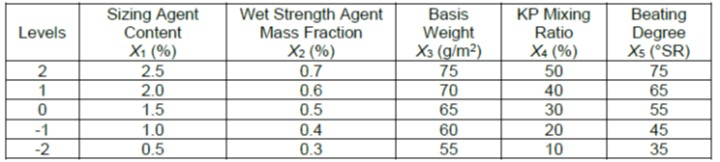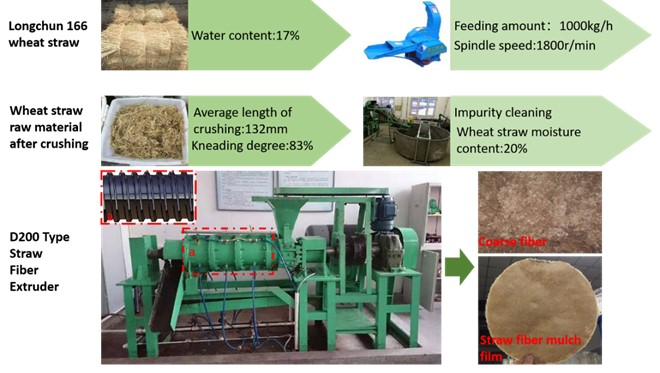Fig. 1. Wheat straw pretreatment process, the manufacturing process uses a screw extrusion processing scheme

RESULTS AND DISCUSSION

Experimental Data

A total of 36 groups of experiments were designed, and the experimental results are shown in Table 3. The regression model analysis of variance is shown in Table 4. Through the analysis of Table 3, it was easy to find that the fit of the quadratic regression models was highly significant. The p-values of all three models were less than 0.01 at the confidence level α = 0.05 with F-values of 12.63 for the dry tensile index, 11.81 for the wet tensile index, and 15.41 for the degradation time, respectively. Therefore, it was very accurate to use the quadratic regression model to describe the effects of each factor as well as to predict y1, y2, and y3. The test results of the quadratic coefficients were used to determine the extent of the effect of the factors on y.

Table 3. Experimental Scheme and Results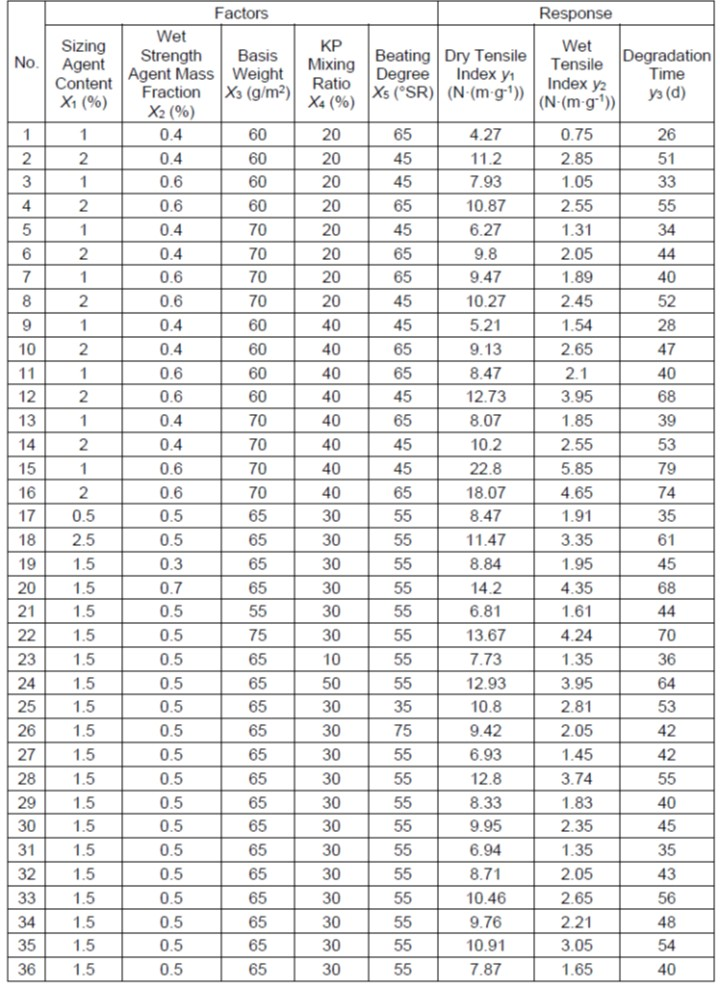Table 4. Variance Analysis of Regression Model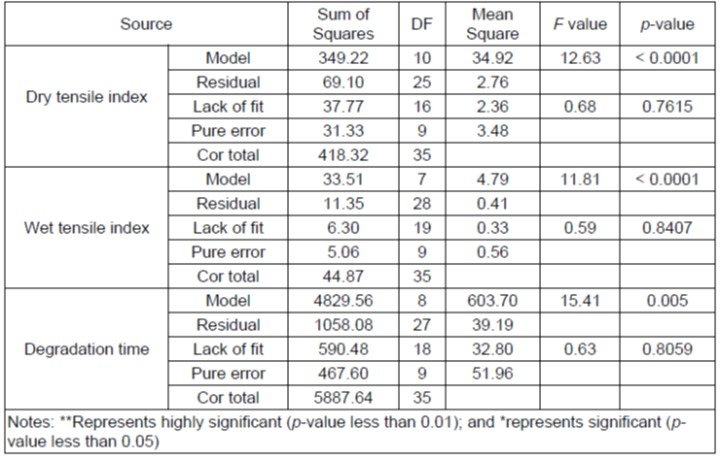According to the analysis of the factors in Table 3. Separate regression models were developed for the dry tensile index (y1), wet tensile index (y2), and degradation time (y3). These models are shown in Eqs. 1 through 3,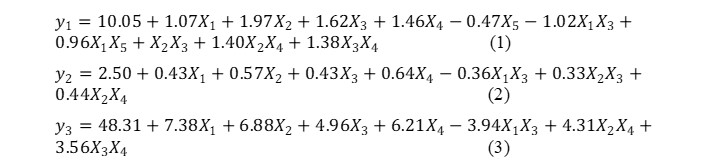where the five influencing factors were included in the formula as follows: the sizing agent content, X1, (%), the wet strength agent mass fraction, X2, (%), the basis weight, X3, (g/m2), the KP mixing ratio, X4, (%), and the beating degree of the wheat straw fiber, X5, (°SR)

Influences of the Tested Factors on the Dry Tensile Index

The effect of the sizing agent content (X1) and basis weight (X3) on the dry tensile index (y1) of the film at level 0 for the other factors is shown in Fig. 2a. At a low sizing agent content, the dry tensile index sharply increased as the basis weight increased, but this trend slowed down as the sizing agent content increased. This was especially true when the sizing agent content was above the 0 level, as the dry tensile index growth trend was not significant. This was primarily because at a lower sizing agent content, with the basis weight increasing, the number of fibers that could be lapped increased, which would enhance the stability of the structure via the effects of colloid curing after drying. Thus, the dry tensile index of the film was improved. However, when the sizing agent content reached saturation, additional sizing agent did not have any further effect on the dry tensile index. As can be seen in Fig. 2a, the basis weight had a similar positive effect on the dry tensile index as the sizing agent content, and the interaction of these two factors also had a positive effect on the dry tensile index. The maximum value occurred at a basis weight of 75 g/m2 and a sizing agent content of 0.50%.

The effect of the sizing agent content (X1) and the beating degree of the wheat straw fiber (X5) on the dry tensile index (y1) at level 0 for the other influencing factors is shown in Fig. 2b. When the sizing agent content was low, the dry tensile index of the film showed a decreasing trend as the beating degree of the wheat straw fiber increased, but this decreasing trend kept slowing down as the sizing agent content increased, and it changed to an increasing trend when the sizing agent content was above 0 level. This was because as the beating degree of the wheat straw fiber increased, the free state of the fiber fraction increased and the amount of fiber branching was less, while a low sizing agent content was not enough to lap all the fibers together. However, when the sizing agent content increased, it would help more free state fibers build together to increase the stability of the structure. As known from the response surface shown in Fig. 2b, the negative effect of the beating degree of the wheat straw fiber on the dry tensile index was less than the positive effect of the sizing agent (X1) when the sizing agent content was located above level 0. The maximum value occurred at a beating degree of the wheat straw fiber of 75 °SR and a sizing agent content of 2.50%.

The effect of the wet strength agent mass fraction (X2) and basis weight (X3) on the effect of the dry tensile index (y1) at level 0 for the other influencing factors is shown in Fig. 2c. The effect of the basis weight on the dry tensile index was not significant at a low level of the wet strength agent mass fraction. As the wet strength agent content increased, the dry tensile index gradually increased, especially when the wet strength agent mass fraction was above 0 level. The wet strength agent could help the structure of the film maintain stability in the wet state, so the dry tensile index would increase as the wet strength agent mass fraction and basis weight increased. As is apparent from the response surface shown in Fig. 2c, both of these two factors had a positive effect on the dry tensile index, and the maximum value was obtained at a basis weight of 75 g/m2 and a wet strength agent mass fraction of 0.7%.

The effect of the wet strength agent mass fraction (X2) and the KP pulp mixing ratio (X4) on the dry tensile index (y1) at level 0 for the other influencing factors is shown in Fig. 2d. At a low wet strength agent mass fraction, the effect of the KP pulp mixing ratio on the dry tensile index was not significant, and the trend effect was gradually increasing as the wet strength agent mass fraction increased, especially when the wet strength agent mass fraction was above level 0, as the increasing trend became significant. Because sulfate pulp had better fiber lap capacity and strength, increasing the KP pulp mixing ratio would increase the strength of the membrane. As known from the response surface shown in Fig. 2d, the combination of the KP pulp mixing ratio and the wet strength agent mass fraction could have a positive effect. The maximum value occurred when the wet strength agent mass fraction was 0.7% and the KP pulp mixing ratio was 50%.

The effect of the basis weight (X3) and the KP mixing ratio (X4) on the dry tensile index (y1) when the other influencing factors were at level 0 is shown in Fig. 2e. When the basis weight was at a low level, the increase in the dry tensile index of the mulch as the KP mixing ratio increased was not significant. When the basis weight was above level 0, the increasing trend of the dry tensile index became more and more dramatic as the KP mixing ratio increased. The reason for this was that a greater KP mixing ratio and basis weight would help to enhance the strength of the mulch film, which could increase the dry tensile index. As known from the response surface shown in Fig. 2e, the combination of the KP mixing ratio and the basis weight could have a positive effect. The maximum value obtained was when the basis weight was 75 g/m2 and the KP mixing ratio was 50%.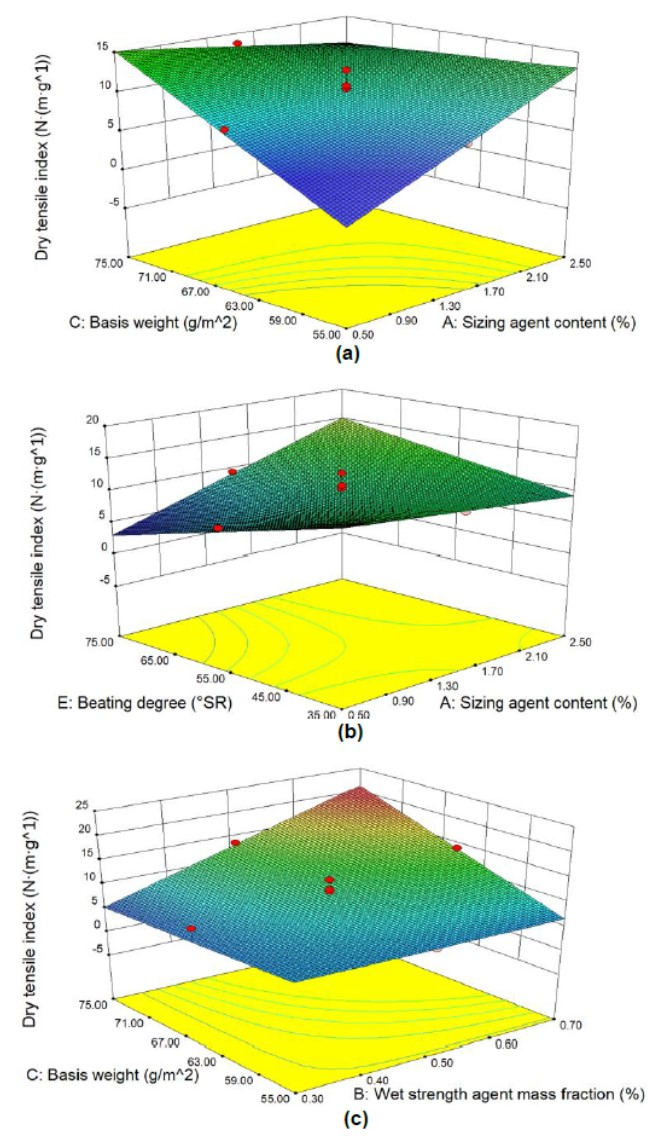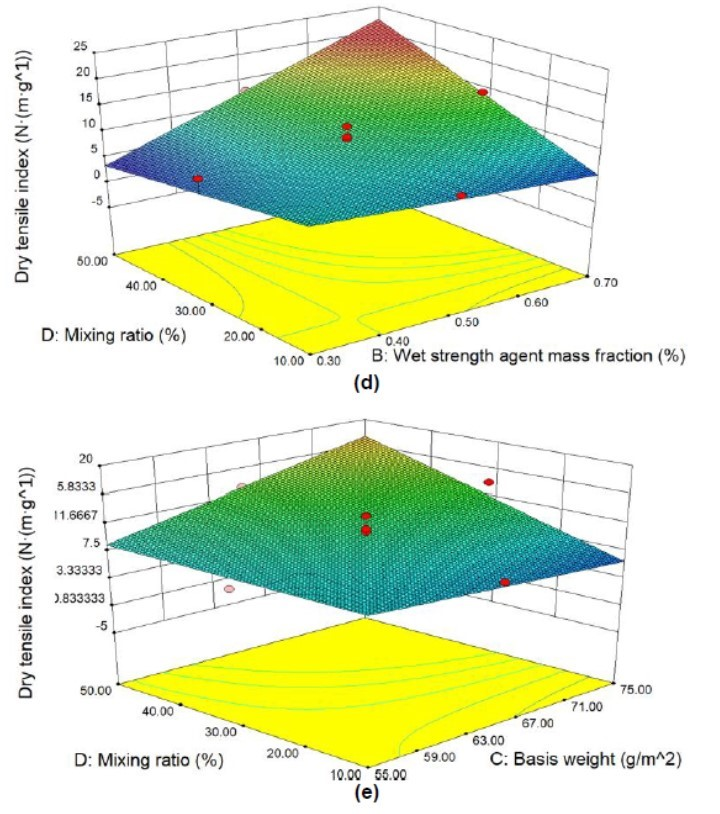Fig. 2. Response surface plots of the factors affecting the dry tensile index: (a) influence of the sizing agent content and the basis weight on the dry tensile index; (b) influence of the beating degree of the wheat straw fiber and the sizing agent content on the dry tensile index; (c) influence of the wet strength agent mass fraction and the basis weight on the dry tensile index; (d) influence of the KP mixing ratio and the wet strength agent mass fraction on the dry tensile index; and (e) influence of the basis weight and the KP mixing ratio on the dry tensile index.

Influences of the Tested Factors on the Wet Tensile Index

The effect of the sizing agent content (X1) and basis weight (X3) on the wet tensile index (y2) of the film at level 0 for the other factors is shown in Fig. 3a. At a lower sizing agent content, the basis weigh exerted a beneficial effect on the wet tensile index, but this trend slowed down as the sizing agent content increased. When the sizing agent content was above level 0, the wet tensile index had a decreasing trend. This was because additional sizing agent and a higher basis weight can enhance the stability of the bonding between the fibers, which would enhance the wet tensile index.